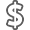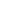# The Basic Practice of Statistics

• ISBN13:

• ISBN10:

## 1319042570

• Edition: 8th
• Format: Hardcover
• Publisher: W. H. Freeman

Note: Supplemental materials are not guaranteed with Rental or Used book purchases.

Purchase Benefits

•Free Shipping On Orders Over \$35!
Your order must be \$35 or more to qualify for free economy shipping. Bulk sales, PO's, Marketplace items, eBooks and apparel do not qualify for this offer.
•Get Rewarded for Ordering Your Textbooks! Enroll Now
•We Buy This Book Back!
In-Store Credit: \$26.25
Check/Direct Deposit: \$25.00
PayPal: \$25.00
List Price: \$269.91 Save up to \$210.08
• Rent Book \$94.47Free Shipping

TERM
PRICE
DUE
IN STOCK USUALLY SHIPS WITHIN 24-48 HOURS.
*This item is part of an exclusive publisher rental program and requires an additional convenience fee. This fee will be reflected in the shopping cart.

### Supplemental Materials

What is included with this book?

• The New copy of this book will include any supplemental materials advertised. Please check the title of the book to determine if it should include any access cards, study guides, lab manuals, CDs, etc.
• The Used, Rental and eBook copies of this book are not guaranteed to include any supplemental materials. Typically, only the book itself is included. This is true even if the title states it includes any access cards, study guides, lab manuals, CDs, etc.

### Summary

Prepare yourself to carry out regular statistical procedures and follow statistical reasoning regardless of your field of study as Basic Practice of Statistics readies you for success in college and beyond.

PART I: EXPLORING DATA

Chapter 0 Getting Started

Where data comes from matters

Always look at the data

Variation is everywhere

What lies ahead in this book

Chapter 1 Picturing Distributions with Graphs

1.1 Individuals and variables

1.2 Categorical variables: Pie charts and bar graphs

1.3 Quantitative variables: Histograms

1.4 Interpreting histograms

1.5 Quantitative variables: Stemplots

1.6 Time plots

Chapter 2 Describing Distributions with Numbers

2.1 Measuring center: The mean

2.2 Measuring center: The median

2.3 Comparing the mean and the median

2.5 The five-number summary and boxplots

2.6 Spotting suspected outliers*

2.7 Measuring spread: The standard deviation

2.8 Choosing measures of center and spread

2.9 Using technology

2.10 Organizing a statistical problem

Chapter 3 The Normal Distributions

3.1 Density curves

3.2 Describing density curves

3.3 Normal distributions

3.4 The 68-95-99.7 rule

3.5 The standard Normal distribution

3.6 Finding Normal proportions

3.7 Using the standard Normal table

3.8 Finding a value given a proportion

Chapter 4 Scatterplots and Correlation

4.1 Explanatory and response variables

4.2 Displaying relationships: Scatterplots

4.3 Interpreting scatterplots

4.4 Adding categorical variables to scatterplots

4.5 Measuring linear association: Correlation

Chapter 5 Regression

5.1 Regression lines

5.2 The least-squares regression line

5.3 Using technology

5.5 Residuals

5.6 Influential observations

5.7 Cautions about correlation and regression

5.8 Association does not imply causation

5.9 Correlation, prediction, and big data*

Chapter 6 Two-Way Tables*

6.1 Marginal distributions

6.2 Conditional distributions

Chapter 7 Exploring Data: Part I Review

Part I Summary

Test Yourself

Supplementary Exercises

PART II: PRODUCING DATA

Chapter 8 Producing Data: Sampling

8.1 Population versus sample

8.3 Simple random samples

8.5 Other sampling designs

8.7 The impact of technology

Chapter 9 Producing Data: Experiments

9.1 Observation versus experiment

9.2 Subjects, factors, treatments

9.4 Randomized comparative experiments

9.5 The logic of randomized comparative experiments

9.7 Matched pairs and other block designs

Chapter 10 Data Ethics*

10.1 Institutional review boards

10.2 Informed consent

10.3 Confidentiality

10.4 Clinical trials

10.5 Behavioral and social science experiments

Chapter 11 Producing Data: Part II Review

Part II summary

Test yourself

Supplementary exercises

PART III: FROM DATA PRODUCTION TO INFERENCE

Chapter 12 Introducing Probability

12.1 The idea of probability

12.2 The search for randomness*

12.3 Probability models

12.4 Probability rules

12.5 Discrete probability models

12.6 Continuous probability models

12.7 Random variables

12.8 Personal probability*

Chapter 13 General Rules of Probability*

13.2 Independence and the multiplication rule

13.3 Conditional probability

13.4 The general multiplication rule

13.5 Showing events are independent

13.6 Tree diagrams

13.7 Bayes' rule*

Chapter 14 Binomial Distributions*

14.1 The binomial setting and binomial distributions

14.2 Binomial distributions in statistical sampling

14.3 Binomial probabilities

14.4 Using technology

14.5 Binomial mean and standard deviation

14.6 The Normal approximation to binomial distributions

Chapter 15 Sampling Distributions

15.1 Parameters and statistics

15.2 Statistical estimation and the law of large numbers

15.3 Sampling distributions

15.4 The sampling distribution of x

15.5 The central limit theorem

15.6 Sampling distributions and statistical significance*

Chapter 16 Confidence Intervals: The Basics

16.1 The reasoning of statistical estimation

16.2 Margin of error and confidence level

16.3 Confidence intervals for a population mean

16.4 How confidence intervals behave

Chapter 17 Tests of Significance: The Basics

17.1 The reasoning of tests of significance

17.2 Stating hypotheses

17.3 P-value and statistical significance

17.4 Tests for a population mean

17.5 Significance from a table*

Chapter 18 Inference in Practice

18.1 Conditions for inference in practice

18.4 Planning studies: Sample size for confidence intervals

18.5 Planning studies: The power of a statistical test*

Chapter 19 From Data Production to Inference: Part III Review

Part III Summary

Review Exercises

Test Yourself

Supplementary Exercises

Chapter 20 Inference about a Population Mean

20.1 Conditions for inference about a mean

20.2 The t distributions

20.3 The one-sample t confidence interval

20.4 The one-sample t test

20.5 Using technology

20.6 Matched pairs t procedures

20.7 Robustness of t procedures

Chapter 21 Comparing Two Means

21.1 Two-sample problems

21.2 Comparing two population means

21.3 Two-sample t procedures

21.4 Using technology

21.5 Robustness again

21.6 Details of the t approximation*

21.7 Avoid the pooled two-sample t procedures*

21.8 Avoid inference about standard deviations*

Chapter 22 Inference about a Population Proportion

22.1 The sample proportion

22.2 Large-sample confidence intervals for a proportion

22.3 Choosing the sample size

22.4 Significance tests for a proportion

22.5 Plus four confidence intervals for a proportion*

Chapter 23 Comparing Two Proportions

23.1 Two-sample problems: Proportions

23.2 The sampling distribution of a difference between proportions

23.3 Large-sample confidence intervals for comparing proportions

23.4 Using technology

23.5 Significance tests for comparing proportions

23.6 Plus four confidence intervals for comparing proportions*

Chapter 24 Inference about Variables: Part IV Review

Part IV summary

Test yourself

Supplementary exercises

Chapter 25 Two Categorical Variables: The Chi-Square Test

25.1 Two-way tables

25.2 The problem of multiple comparisons

25.3 Expected counts in two-way tables

25.4 The chi-square test statistic

25.5 Using technology

25.6 The chi-square distributions

25.7 Cell counts required for the chi-square test

25.8 Uses of the chi-square test: Independence and homogeneity

25.9 The chi-square test for goodness of fit*

Chapter 26 Inference for Regression

26.1 Conditions for regression inference

26.2 Estimating the parameters

26.3 Using technology

26.4 Testing the hypothesis of no linear relationship

26.5 Testing lack of correlation

26.6 Confidence intervals for the regression slope

26.8 Checking the conditions for inference

Chapter 27 One-Way Analysis of Variance:

Comparing Several Means

27.1 Comparing several means

27.2 The analysis of variance F test

27.3 Using technology

27.4 The idea of analysis of variance

27.5 Conditions for ANOVA

27.6 F distributions and degrees of freedom

27.7 Follow-up analysis: Tukey pairwise multiple comparisons

27.8 Some details of ANOVA*

[Back matter print text]

Notes and Data Sources

Tables

TABLE A Standard Normal probabilities

TABLE B Random digits

TABLE C t distribution critical values

TABLE D Chi-square distribution critical values

TABLE E Critical values of the correlation r

Index

PART VI: OPTIONAL COMPANION CHAPTERS

(available online)

Chapter 28 Nonparametric Tests

28.1 Comparing two samples: The Wilcoxon rank sum test

28.2 The Normal approximation for W

28.3 Using technology

28.4 What hypotheses does Wilcoxon test?

28.5 Dealing with ties in rank tests

28.6 Matched pairs: The Wilcoxon signed rank test

28.7 The Normal approximation for W+

28.8 Dealing with ties in the signed rank test

28.9 Comparing several samples: The Kruskal-Wallis test

28.10 Hypotheses and conditions for the Kruskal-Wallis test

28.11 The Kruskal-Wallis test statistic

Chapter 29 Multiple Regression

29.1 Parallel regression lines

29.2 Estimating parameters

29.3 Using technology

29.4 Inference for multiple regression

29.5 Interaction

29.6 The multiple linear regression model

29.7 The woes of regression coefficients

29.8 A case study for multiple regression

29.9 Inference for regression parameters

29.10 Checking the conditions for inference

Chapter 30 More about Analysis of Variance

30.1 Beyond one-way ANOVA

30.2 Two-way ANOVA: Conditions, main effects, and interaction

30.3 Inference for two-way ANOVA

30.4 Some details of two-way ANOVA*

Chapter 31 Statistical Process Control

31.1 Processes

31.2 Describing processes

31.3 The idea of statistical process control

31.4 x charts for process monitoring

31.5 s charts for process monitoring

31.6 Using control charts

31.6 Setting up control charts

31.8 Don't confuse control with capability!

31.9 Control charts for sample proportions

31.10 Control limits for p charts

Chapter 32 Resampling: Permutation Tests and the Bootstrap

32.1 Randomization in experiments as a basis for inference

32.2 Permutation tests for comparing two treatments with software

32.3 Generating bootstrap samples

32.4 Bootstrap standard errors and confidence intervals